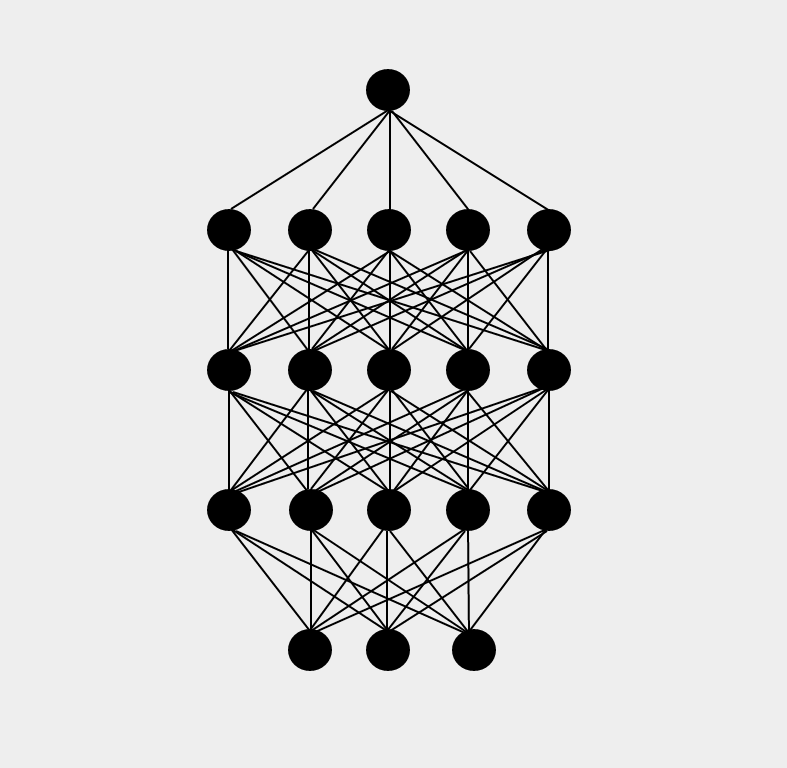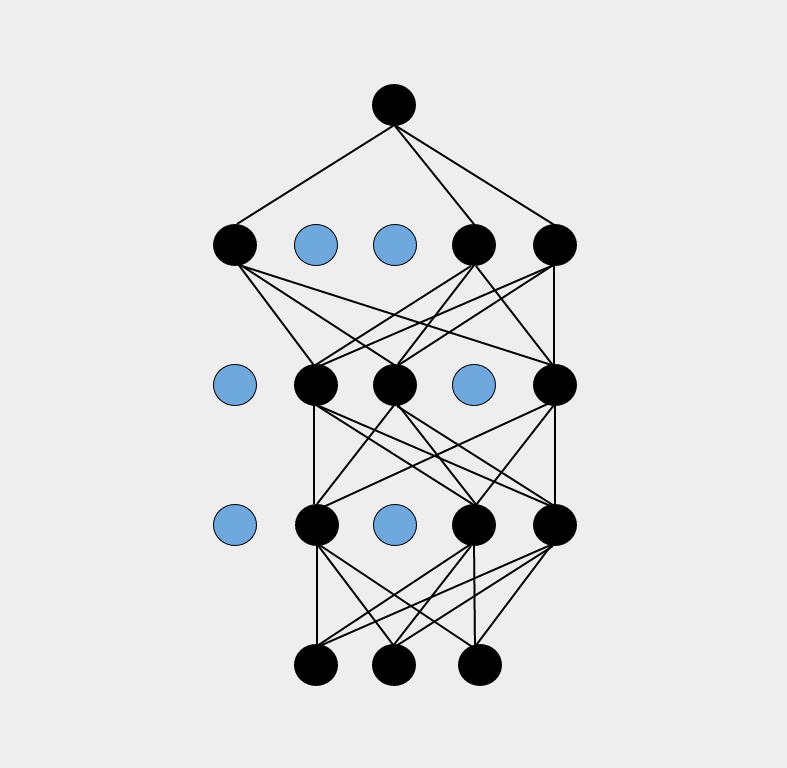# Dropout with Theano

Deep LearningModelingPythonToolsTools & LanguagesPython|Theanoposted by Rishabh Shukla April 2, 2017

Almost everyone working with Deep Learning would have heard a smattering about Dropout. Albiet a simple concept (introduced a couple of...Almost everyone working with Deep Learning would have heard a smattering about Dropout. Albiet a simple concept (introduced a couple of years ago), which sounds like a pretty obvious way for model averaging, further resulting into a more generalized and regularized Neural Net; still when you actually get into the nitty-gritty details of implementing it in your favorite library (theano being mine), you might find some roadblocks there. Why? Because it’s not exactly straight-forward to randomly deactivate some neurons in a DNN.

In this post, we’ll just recapitulate what has already been explained in detail about Dropout in lot of papers and online resources(some of these are provided at the end of the post). Our main focus will be on implementing a Dropout layer in Numpy and Theano, while taking care of all the related caveats. You can find the Jupyter Notebook with the Dropout Class here.

Regularization is a technique to prevent Overfitting in a machine learning model. Considering the fact that a DNN has a highly complex function to fit, it can easily overfit with a small/intermediate size of dataset.

In very simple terms – Dropout is a highly efficient regularization technique, wherein, for each iteration we randomly remove some of the neurons in a DNN(along with their connections; have a look at Fig. 1). So how does this help in regularizing a DNN? Well, by randomly removing some of the cells in the computational graph(Neural Net), we are preventing some of the neurons(which are basically hidden features in a Neural Net) from overfitting on all of the training samples. So, this is more like just considering only a handful of features(neurons) for each training sample and producing the output based on these features only. This results into a completely different neural net(hopefully ;)) for each training sample, and eventually our output is the average of these different nets(any `Random Forests`-phile here? :D).

## Graphical Overview:

In Fig. 1, we have a fully connected deep neural net on the left side, where each neuron is connected to neurons in its upper and lower layers. On the right side, we have randomly omitted some neurons along with their connections. For every learning step, Neural net in Fig. 2 will have a different representation. Consequently, only the connected neurons and their weights will be learned in a particular learning step.Fig. 1 – Left: DNN without Dropout, Right: DNN with some dropped neurons

## Theano Implementation:

Let’s dive straight into the code for implementing a Dropout layer. If you don’t have prior knowledge of Theano and Numpy, then please go through these two awesome blogs by @dennybritzImplementing a Neural Network from Scratch and Speeding up your neural network with theano and gpu.

As recommended, whenever we are dealing with Random numbers, it is advisable to set a random seed.

Let’s enumerate through each line in the above code. Firstly, we import all the necessary modules(more about `RandomStreams` in the next few lines) and initialize the random seed, so the random numbers generated are consistent in each different run. On the second line we create an object `rng` of `numpy.random.RandomState`, this exposes a number of methods for generating random numbers, drawn from a variety of probability distributions.

Theano is designed in a functional manner, as a result of this generating random numbers in Theano Computation graphs is a bit tricky compared to Numpy. Using Random Variables with Theano is equivalent to imputing random variables in the Computation graph. Theano will allocate a numpy `RandomState` object for each such variable, and draw from it as necessary. Theano calls this sort of sequence of random numbers a `Random Stream`. The `MRG_RandomStreams` we are using is another implementation of `RandomStreams` in Theano, which works for GPUs as well.

So, finally we create a `srng` object which will provide us with Random Streams in each run of our Optimization Function.

Here is our main Dropout function with three arguments: `srng` – A RandomStream generator, `weight` – Any theano tensor(Weights of a Neural Net), and `drop` – a float value to denote the proportion of neurons to drop. So, naturally number of neurons to retain will be `1 - drop`.

On the second line in the function, we are generating a RandomStream from Binomial Distribution, where `n` denotes the number of trials, `p` is the probability with which to retain the neurons and `size` is the shape of the output. As the final step, all we need to do is to switch the value of some of the neurons to `0`, which can be accomplished by simply multiplying `mask` with the `weight` tensor/matrix. `theano.tensor.cast` is further type casting the resulting value to the value of `theano.config.floatX`, which is either the default value of `floatX`, which is `float32` in theano or any other value that we might have mentioned in `.theanorc` configuration file.

Now, one thing to keep in mind is – we only want to drop neurons during the training phase and not during the validation or test phase. Also, we need to somehow compensate for the fact that during the training time we deactivated some of the neurons. There are two ways to achieve this:

1. Scaling the Weights(implemented at the test phase): Since, our resulting Neural Net is an averaged model, it makes sense to use the averaged value of the weights during the test phase, considering the fact that we are not deactivating any neurons here. The easiest way to do this is to scale the weights(which acts as averaging) by the factor of retained probability, in the training phase. This is exactly what we are doing in the above function.
2. Inverted Dropout(implemented at the training phase): Now scaling the weights has its caveats, since we have to tweak the weights at the test time. On the other end ‘Inverted Dropout’ performs the scaling at the training time. So, we don’t have to tweak the test code whenever we decide to change the order of Dropout layer. In this post, we’ll be using the first method(scaling), although I’d recommend you to play with Inverted Dropout as well. You can follow this up for the guidance.

Our final `dropout_layer` function uses `theano.ifelse` module to return the value of either `dropit` or `dont_dropit` function. This is conditioned on whether our `train` flag is on or off. So, while the model is in training phase, we’ll use dropout for our model weights and in test phase, we would simply scale the weights to compensate for all the training steps, where we omitted some random neurons.

Finally, here’s how you can add a Dropout layer in your DNN. I am taking an example of RNN, similar to the one used in this blog:

Here, we have the `forward_prop` function for RNN+GRU model. Starting from the first line, we are creating a theano tensor variable `x`, for input(words) and another `drop_value` variable of type `theano.tensor.scalar`, which will take a float value to denote the proportion of neurons to be dropped.

Then we are creating an object `dropout` of the `Dropout` class, we implemented in previous sections. After this, we are initiating a `GRU` object(I have kept this as a generic class, since you might have a different implementation). We also have one more variable, namely `params` which is an `OrderedDict` containing the model parameters.

Furthermore, `E` is our Word Embedding Matrix, `U` contains, input to hidden layer weights, `W` is the hidden to hidden layer weights and `b` is the bias. Then we have our workhorse – the `forward_prop` function, which is called iteratively for each value in `x`variable(here these values will be the indexes for sequential words in the text). Now, all we have to do is call the `dropout_layer` function from `forward_prop`, which will return `W`, `U`, with few dropped neurons.

This is it in terms of implementing and using a dropout layer with Theano. Although, there are a few things mentioned in the next section, which you have to keep in mind when working with `RandomStreams`.

## Few things to take care of:

Wherever we are going to use a `theano.function` after this, we’ll have to explicitly pass it the `updates`, we got from `theano.scan` function in previous section. Reason?Whenever there is a call to theano’s `RandomStreams`, it throws some updates, and all of the theano functions, following the above code, should be made aware of these updates. So let’s have a look at this code:

As a standard procedure, we are using another model parameter `V`(hidden to output) and taking a `softmax` over this. If you have a look at `predict`, `loss` functions, then we had to explicitly, tell them about the `updates` that `RandomStreams` made during the execution of `dropout_layer` function. Else, this will throw an error in Theano.

What is the appropriate float value for dropout? To be on the safe side, a value of `0.5`(as mentioned in the original paper) is generally good enough. Although, you could always try to tweak it a bit and see what works best for your model.

## Alternatives to Dropout

Lately, there has been a lot of research for better regularization methods in DNNs. One of the things that I really like about Dropout is that it’s conceptually very simple as well as an highly effective way to prevent overfitting. A few more methods, that are increasingly being used in DNNs now a days(I am omitting the standard L1/L2 regularization here):

1. Batch Normalization: Batch Normalization primarily tackles the problem of internal covariate shift by normalizing the weights in each mini-batch. So, in addition to simply using normalized weights at the beginning of the training process, Batch Normalization will keep on normalizing them during the whole training phase. This accelerates the optimization process and as a side product, might also eliminate the need of Dropout. Have a look at the original paper for more in-depth explanation.
2. Max-Norm: Max-Norm puts a specific upper bound on the magnitude of weight matrices and if the magnitude exceeds this threshold then the values of weight matrices are clipped down. This is particularly helpful for exploding gradient problem.
3. DropConnect: When training with Dropout, a randomly selected subset of activations are set to zero within each layer. DropConnect instead sets a randomly selected subset of weights within the network to zero. Each unit thus receives input from a random subset of units in the previous layer. We derive a bound on the generalization performance of both Dropout and DropConnect. – Abstract from the original paper.
4. ZoneOut(specific to RNNs): In each training step, ZoneOut keeps the value of some of the hidden units unchanged. So, instead of throwing out the information, it enforces a random number of hidden units to propogate the same information in next time step.

The reason I wanted to write about this, is because if you are working with a low level library like Theano, then sometimes using modules like `RandomStreams` might get a bit tricky. Although, for prototyping and even for production purposes, you should also consider other high level libraries like Keras and TensorFlow.

Feel free, to add any other regularization methods and feedbacks, in the comments section.